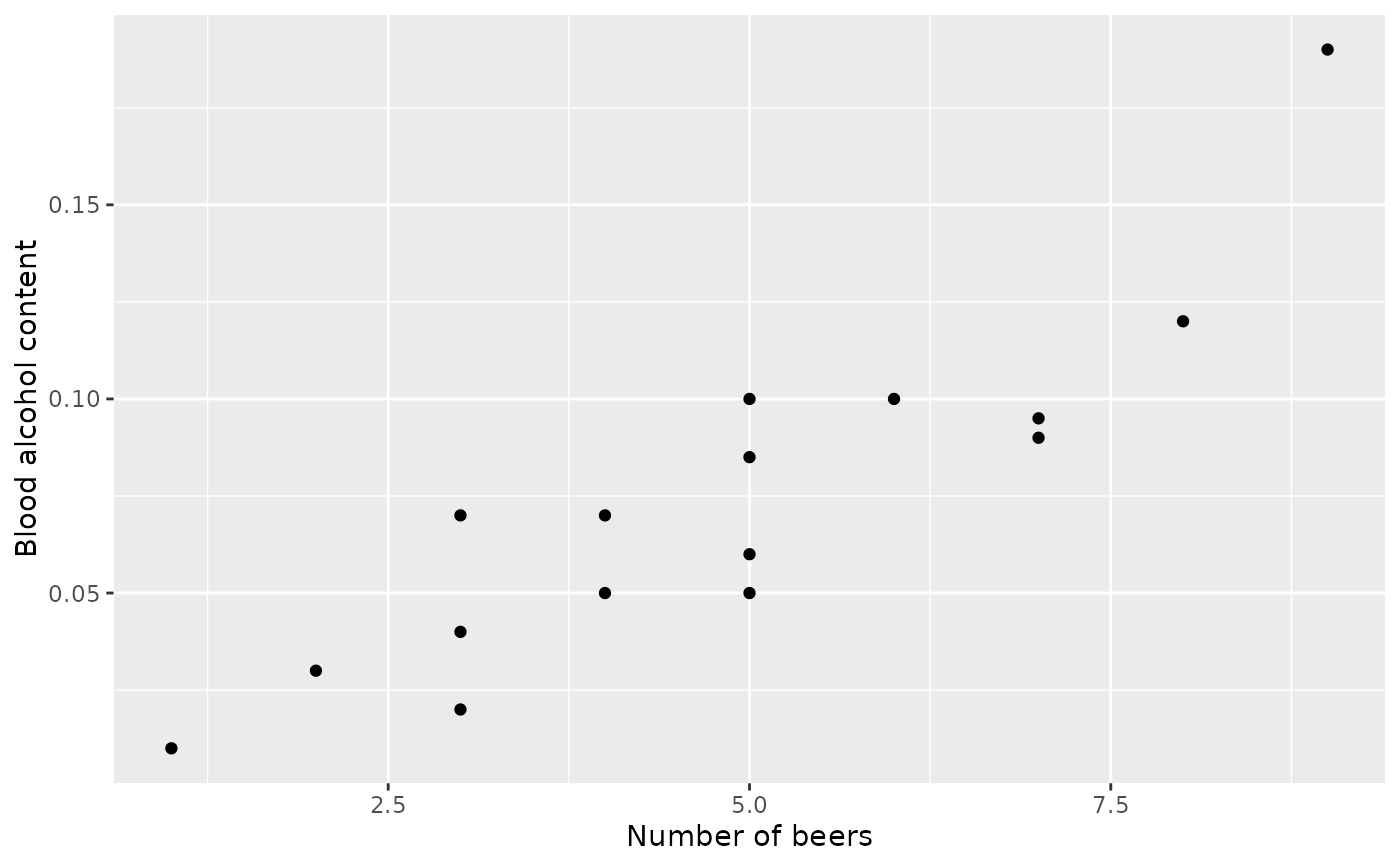Here we examine data from sixteen student volunteers at Ohio State University who each drank a randomly assigned number of cans of beer.

## Usage

bac

## Format

A data frame with 16 observations on the following 3 variables.

student

a numeric vector

beers

a numeric vector

bac

a numeric vector

## Source

J. Malkevitch and L.M. Lesser. For All Practical Purposes: Mathematical Literacy in Today's World. WH Freeman & Co, 2008.

## Examples


library(ggplot2)

ggplot(bac, aes(x = beers, y = bac)) +
geom_point() +
labs(x = "Number of beers", y = "Blood alcohol content")